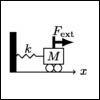18.03SC | Fall 2011 | Undergraduate

# Differential Equations

Unit II: Second Order Constant Coefficient Linear Equations

## Modes and the Characteristic Equation

« Previous | Next »

### Session OverviewWe now begin an in depth study of constant coefficient linear equations. These are the most important DE’s in 18.03, and we will be studying them up to the last few sessions. In this session we will learn algebraic techniques for solving these equations. Exponential functions will play a major role and we will see that higher order linear constant coefficient DE’s are similar in many ways to the first order equation x’ + kx = 0.

### Session Activities

Watch the lecture video clips:

Take the quiz:

### Session Activities

Watch the lecture video clips:

Watch the lecture video clip:

Watch the problem solving videos:

Complete the practice problems:

### Check Yourself

Complete the problem set:

« Previous | Next »

## Course Info

Fall 2011
##### Learning Resource Types
Lecture Videos
Recitation Videos
Exams with Solutions
Simulations
Lecture Notes
Problem Sets with Solutions